NEET  >  Test: Chemistry - 1

# Test: Chemistry - 1 - NEET

Test Description

## 45 Questions MCQ Test NEET Mock Test Series - Test: Chemistry - 1

Test: Chemistry - 1 for NEET 2023 is part of NEET Mock Test Series preparation. The Test: Chemistry - 1 questions and answers have been prepared according to the NEET exam syllabus.The Test: Chemistry - 1 MCQs are made for NEET 2023 Exam. Find important definitions, questions, notes, meanings, examples, exercises, MCQs and online tests for Test: Chemistry - 1 below.
Solutions of Test: Chemistry - 1 questions in English are available as part of our NEET Mock Test Series for NEET & Test: Chemistry - 1 solutions in Hindi for NEET Mock Test Series course. Download more important topics, notes, lectures and mock test series for NEET Exam by signing up for free. Attempt Test: Chemistry - 1 | 45 questions in 45 minutes | Mock test for NEET preparation | Free important questions MCQ to study NEET Mock Test Series for NEET Exam | Download free PDF with solutions
 1 Crore+ students have signed up on EduRev. Have you?
Test: Chemistry - 1 - Question 1

### Two oxides of metal contain 27.6℅ and 30℅ oxygen respectively. If formula of first oxide is M3O4, then formula of second oxide is:

Detailed Solution for Test: Chemistry - 1 - Question 1

Given that, formula of first oxide = M3O4
Let mass of the metal = x
% of metal in M3O4 = (3x / 3x + 64) * 100
but as given % age = (100 - 27.6) = 72.4%
so, (3x / 3x + 64) * 100 = 72.4
or x = 56.
in 2nd oxide,
oxygen = 30%....so metal = 70%
so, the ratio is
M : O
70 / 56 : 30 / 16
1.25 : 1.875
2 : 3
so, 2nd oxide is M2O3

Test: Chemistry - 1 - Question 2

### 5.6 litres of a gas at N.T.P. are found to have a mass of 11 g. The molecular mass of the gas is

Detailed Solution for Test: Chemistry - 1 - Question 2

5.6lit = 11g
22.4lit = ? (x) g
x = 22.4 × 11 / 5.6
x = 44g
Thus, Molecular mass of gas is 44g

Test: Chemistry - 1 - Question 3

### The crystalline salt, Na2SO4xH2O on heating loses 55.9% of its weight. The formula of the crystalline salt is

Detailed Solution for Test: Chemistry - 1 - Question 3

Molar mass of salt = (2 × 23) + 32 + (16 × 4) + x × 18
= 142 + 18x
On heating it will lose water and become anhydrous. 55.9% mass is the mass of water in Na2SO4.xH2O.
Mass by mass% = (mass / molar mass of compound) × 100
mass of water = 18x
55.9 = (18x / 142 + 18x) × 100
55.9(142 + 18x) = 1800x
7937.8 + 1006.2x = 1800x
x = 9.99
x = 10 (approx.)
So, the molecular formula of compound is Na2SO4.10H2O.

Test: Chemistry - 1 - Question 4

0.5 g of a metal on oxidation gave 0.79 g of its oxide. The equivalent weight of the metal is (approximately)

Detailed Solution for Test: Chemistry - 1 - Question 4

Given,

Mass of metal = 0.5 g

Mass of oxide = 0.79 g

First we have to calculate the Mass of Oxygen in metal oxide.

Mass of Oxygen = Mass of oxide - Mass of Metal = 0.79 - 0.5 = 0.29 g

The equivalent weight of oxygen is 8 g/eq.

0.29 g of oxygen combines with the 0.5 g of metal

8 g of oxygen combines with  of metal.

Therefore, the equivalent weight of metal is 13.793 g/eq.

Test: Chemistry - 1 - Question 5

Air contains nearly 20% oxygen by volume. The volume of air needed for complete combustion of 100 cc of acetylene will be

Detailed Solution for Test: Chemistry - 1 - Question 5

Equation
2 C2H2 + 5O2 → 4CO2 + 2 H2O
In this reaction, we can see that, 2 moles of C2H2reacts with 5 moles of O2​
Or, 1 mole of ​C2H2 reacts with 5 / 2 moles of O2​
Under standard conditions, we can say that:
22.44 L of C2H2 reacts with (5 / 2) x 22.4 L moles of O2
So, 100 cc of C2H2 will reacts with (5 / 2) x 100 cc = 250 cc of O2​ .
But, since air contains 20 % of oxygen by volume, the amount of air needed to react with 100 cc of C2H2 will be = 250 x (100 / 20) = 1250 cc of air.

Test: Chemistry - 1 - Question 6

A solution of 10 ml(M/10) FeSO4 was titrated with KMnO4 solution in acidic medium. The amount of  KMnO4 used will be

Detailed Solution for Test: Chemistry - 1 - Question 6

The equation of the Mn2+ in acidic medium  will be.

MnO4- + 8H++ 5 Fe2+ → Mn2++ 5Fe3+ +4H2O.

We can balance the reaction by using redox balancing of acid and base.

So, we know from the equation that 5 moles of iron react to form 1 mole of Mn+2.

Thus, we can say that (10*0.1) moles of iron will react with the (10*0.1/5) moles of Manganese ions, which will be 0.2 thus, 10ml volume of KMnO4 and 0.02M moles of KMnO4.  We will get the same result if we use M1V1 = M2V2 formulae.

Test: Chemistry - 1 - Question 7

At S.T.P. the density of CCl4 vapour in g/L will be nearest to

Detailed Solution for Test: Chemistry - 1 - Question 7

Molecular Weight of CCl₄ = 35.5 + 12 x 4 = 154
1 mole CCl₄ vapour=154 g
According to Avogadro’s hypothesis we know that the molar volume of a gas at STP is 22.4 litres
= 154 / 22.4 = 6.87

Test: Chemistry - 1 - Question 8

The law of multiple proportions was proposed by

Detailed Solution for Test: Chemistry - 1 - Question 8

Dalton's law of multiple proportions is part of the basis for modern atomic theory, along with Joseph Proust's law of definite composition (which states that compounds are formed by defined mass ratios of reacting elements) and the law of conservation of mass that was proposed by Antoine Lavoisier.

Test: Chemistry - 1 - Question 9

The anode rays were discovered by

Detailed Solution for Test: Chemistry - 1 - Question 9

The discovery of protons can be attributed to Rutherford. In 1886 Goldstein discovered existence of positively charged rays in the discharge tube by using perforated cathode. These rays were named as anode rays or cannal rays.

Test: Chemistry - 1 - Question 10

Which of the following transitions of electrons in the hydrogen, atom will emit maximum energy ?

Detailed Solution for Test: Chemistry - 1 - Question 10

Energy is given by formula
E = hc / λ
1 / λ = RZ2 [ 1 / n21 - 1 / n22 ]
E = RZ2 hc [ 1 / n21 - 1 / n22]
This energy will be highest when the transition is from n3 → n2.

Test: Chemistry - 1 - Question 11

The uncertainity found from the uncertainity principle (Δx.Δp = h / 4π) is

Detailed Solution for Test: Chemistry - 1 - Question 11

Δx.Δp ≧ h / 4π
So by the equation given in the question, the uncertainity value will be the least.

Test: Chemistry - 1 - Question 12

The number of orbitals in any shell (n) is equal to

Detailed Solution for Test: Chemistry - 1 - Question 12

Maximum number of electrons can be accommodated in a shell of principal quantum number n is 2n2. Since each orbital can accommodate only 2 electrons, maximum number of orbitals =2n2/2= n2

Test: Chemistry - 1 - Question 13

The maximum number of 4f electrons having spin quantum number (-1/2) is

Detailed Solution for Test: Chemistry - 1 - Question 13

Total no. of electrons in F- subshell = 14 (when we include both spin +1/2  & - 1/2)
But maximum no. of electrons = 7 if only one spin either - 1/2 or +1/2.

Test: Chemistry - 1 - Question 14

The number of unpaired electrons in Cu+  (Z= 29) is

Detailed Solution for Test: Chemistry - 1 - Question 14

Answer : The electronic configuration of Cu is,

1s22s22p63s23p64s13d10

The reason for abnormal electronic configuration of Cu is because of the half-filled and fully-filled configuration are the more stable configuration. In this configuration, the d-subshell is fully-filled and s-subshell is half-filled which makes the Cu more stable.

Now, for getting a positive charge, it has to lose 1 electron which will be removed from the outermost 4s orbital and hence all the remaining electrons will be paired leading to 0 unpaired electrons.

Test: Chemistry - 1 - Question 15

The electrons present in K-shell of the atom will differ in

Detailed Solution for Test: Chemistry - 1 - Question 15

Two electrons in K-shell will differ in spin quantum number.
They will have same values for the principal quantum number, azimuthal quantum number, and magnetic quantum number.

For first electron, n = 1,l = 0,m = 0,s = +1 ​/ 2
For second electron, n = 1,l = 0,m = 0,s = −1 / 2​

Test: Chemistry - 1 - Question 16

Consider the following statements. The d-orbitals have

I. four lobes and two nodes

II. four lobes and one node

Which of the above statements are correct ?

Detailed Solution for Test: Chemistry - 1 - Question 16

Total no of angular nodes = l (azimuthal quantum no), so l=2 in d orbital, therefore number of nodes is 2 and it is a fact that opposite lobes have same signs and adjacent ones have opposite signs. there are 4 lobes in d orbital.

Test: Chemistry - 1 - Question 17

Which of the following substances has the lowest boiling point?

Detailed Solution for Test: Chemistry - 1 - Question 17

Boiling Point is directly proportional to VanderWaals forces of attraction. Down the group, this forces increases. so force of attraction in CHF3 is weaker than CHL3, that's why boiling point of CHF3 is lowest.

Test: Chemistry - 1 - Question 18

Which of the following is solid with highest melting point ?

Detailed Solution for Test: Chemistry - 1 - Question 18

SiO2 is a covalent 'network' solid. So the energy required to break bonds will be higher. Therefore the melting point will be higher.

Test: Chemistry - 1 - Question 19

Out of the two compounds shown below, the vapour pressure of B at a particular temperature is expected to be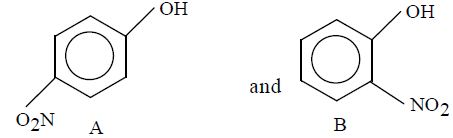Detailed Solution for Test: Chemistry - 1 - Question 19

Due to intramolecular H-bonding  in o-nitrophenol it exists as a monomer while due to intermolecular H-bonding in p-nitrophenol it exists as an associate molecule. As a result, vapour pressure of a o-nitrophenol is higher than that of a p-nitrophenol.

Test: Chemistry - 1 - Question 20

In which of the following molecules, the central atom does not have sp3 hybridisation?

Detailed Solution for Test: Chemistry - 1 - Question 20

When the number of hybrid orbitals, H  is 4, the hybridization is sp3 hybridization
H = 1 / 2[V + M - C + A]
where, V = number of monovalent atoms
C = total positive charge
A = negative charge
a) For CH4
H = 1 / 2[4 + 4 - 0 + 0] = 4
sp3
b) For SF4,
H = 1 / 2 [6 + 4 - 0 + 0] = 5
sp3d
c) For BF-4,
H = 1 / 2 [3 + 4 - 0 + 1] = 4, thus sp3
d) For NH+4
H = 1 / 2[5 + 4 - 1 + 0] = 4 thus sp3
Thus, only in SF4 the central atom does not have sp3 hybridisation.

Test: Chemistry - 1 - Question 21

The correct oreder of increasing bond angles in the following species

Detailed Solution for Test: Chemistry - 1 - Question 21

According to VESPR theory repulsion order

lp - lp > lp - bp > bp - bp

As the number of lone pairs of electrons increases, bond angle decreases due to repulsion between lp-lp, Moreover, as the electronegativity of central atom decreases, bond angle decreases.
The correct order of increasing bond angle is Cl2O < ClO2- < ClO2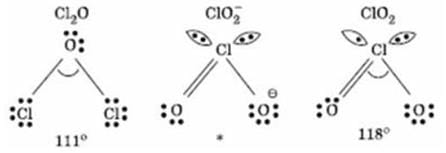In ClO2- there are 2 Ione pairs of electrons present on the central chlorine atom. Therefore the bond angle in ClO2is less than 118° which is the bond angle in ClO2 which has less number of electrons on chlorine.

Test: Chemistry - 1 - Question 22

The correct order of increasing covalent character of the following is

Detailed Solution for Test: Chemistry - 1 - Question 22

Option b is correct  because as the charge increases covalent character also increases.

Test: Chemistry - 1 - Question 23

If Z-axis is the molecular axis, then π molecular orbitals are formed by the overlap of

Detailed Solution for Test: Chemistry - 1 - Question 23

px-px and py-py will form pi bond when z-axis is molecular axis as the options have only one of these so option (d) is the correct answer.

Test: Chemistry - 1 - Question 24

The ionic species having largest size is

Detailed Solution for Test: Chemistry - 1 - Question 24

Li+ have larger size in aq.solution due to the presence of the water molecules present inside the solution surronds easily and become the solution to be more hydrated comparing to the corresponding element present in the aqeous solution.

Test: Chemistry - 1 - Question 25

From the following species, which one is the easiest to remove one electron ?

Detailed Solution for Test: Chemistry - 1 - Question 25

Ionisation Potential is directly proportional to positive oxidation state and inversely proportional to negative oxidation state.So, in O2- removal of electron is easiest.

Test: Chemistry - 1 - Question 26

Electron gain enthalpy of inert gases is

Detailed Solution for Test: Chemistry - 1 - Question 26

On moving down the group, there is an increase in the size of atoms. As a result, the tendency to accept an additional electron decreases and hence, the electron gain enthalpy decreases from Ne to Rn. However, He has small size and much higher tendency to accept an additional electron. Hence, its electron gain enthalpy is least positive of all the noble gases.

Thus, it is concluded that neon has the highest positive electrons gain enthalpy.

Test: Chemistry - 1 - Question 27

The electron gain enthalpies of B,C,N and O are in the order

Detailed Solution for Test: Chemistry - 1 - Question 27

Actually electron gain enthalphy and ionisation energy both are dependent on electronic configuration.
Electronegativity means tendency to attract electron it doesnot matter where it will keep the electron
Electron gain entalphy basically refer to attract e it must have space to keep electron in sub shell like spdf should be present
order of electronegativity is
F > O > N > Cl > Br  > I > S > C > P

Test: Chemistry - 1 - Question 28

With respect to chlorine, hydrogen will be

Detailed Solution for Test: Chemistry - 1 - Question 28

With respect to Chlorine hydrogen will be electropositive. Chlorine being a strong electronegative element tends to force hydrogen to loss electron and hydrogen acquire electropositive character so that chlorine can complete its octet .

Test: Chemistry - 1 - Question 29

Which element has the greatest tendency to lose electrons ?

Detailed Solution for Test: Chemistry - 1 - Question 29

Francium has the greatest tendency to lose electrons.

Test: Chemistry - 1 - Question 30

Which of the following atomic numbers represents an alkali metals ?

Detailed Solution for Test: Chemistry - 1 - Question 30

37 = Rb
55 = Cs
87 = Fr
All belong to alkali metal group.

Test: Chemistry - 1 - Question 31

LiNO3 on heating gives

Detailed Solution for Test: Chemistry - 1 - Question 31

Lithium nitrate when heated decomposes to give lithium oxide and nitrogen dioxide. The reaction is as follows :

4LiNO₃ → 2Li₂O+ 4NO₂+ O₂

LiNO₃ on heating, gives reddish brown fumes of NO₂

Test: Chemistry - 1 - Question 32

Which of the following is an ore of potassium ?

Detailed Solution for Test: Chemistry - 1 - Question 32

Carnallite is an ore of potassium i.e., KCl.MgCl2.6H2O.

Test: Chemistry - 1 - Question 33

Which of the following decomposes at highest temperature ?

Detailed Solution for Test: Chemistry - 1 - Question 33

BaCO3 decomposes at highest temp.

All the carbonates decompose on heating to give CO2 and metal oxide.The stability of carbonate towards heat depends upon the stability of the resulting metal oxide. More is the stability of the resulting metal oxide lesser is the stability of the carbonate towards heat and vice versa.

Test: Chemistry - 1 - Question 34

Nitrolim which is used as a fertilizer has the composition

Detailed Solution for Test: Chemistry - 1 - Question 34

Calcium cyanamide (CaCN2) mixed with carbon (C) is called nitrolim.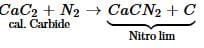Test: Chemistry - 1 - Question 35

The difference of water molecules in gypsum and plaster of paris is

Detailed Solution for Test: Chemistry - 1 - Question 35

Gypsum is CaSO4.2H2O and Plaster of Paris is CaSO4.1 / 2H2O. Difference in number of water molecules = 3 / 2 or 1 x 1 / 2

Test: Chemistry - 1 - Question 36

Which of the following does not contain fused benzene rings ?

Detailed Solution for Test: Chemistry - 1 - Question 36

In Diphenyl the two Benzene rings share a carbon -carbon bond but not the two carbon atoms.

Test: Chemistry - 1 - Question 37

The IUPAC name of the compound,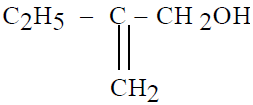is

Detailed Solution for Test: Chemistry - 1 - Question 37

As the double bond has to be taken in main chain and also the carbon to with functional group has to be numbered first thus option A is the correct one.

Test: Chemistry - 1 - Question 38

IPUAC name of the compound,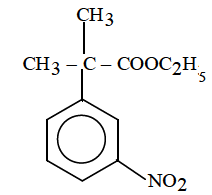Detailed Solution for Test: Chemistry - 1 - Question 38

The IUPAC name of the given compound  is Ethyl-2-methyl-2-(3-nitrophenyl) propanoate,because the side chain contains the functional group , then the phenyl ring is considered as a substituent.

Test: Chemistry - 1 - Question 39

The total number of structural isomers (acyclic and cyclic) possible for the molecular formula, C6H6 are

Detailed Solution for Test: Chemistry - 1 - Question 39

1 linear and 1 cyclic benzene ring.

Test: Chemistry - 1 - Question 40

Which of the following does not show tautomerism ?

Detailed Solution for Test: Chemistry - 1 - Question 40

For an aldehyde or a ketone to exhibit tautomerism it is essential that it must have at least one α−Hα−H atom.

So, C6H5COC(CH3)3

Hence D is the correct answer.

Test: Chemistry - 1 - Question 41

The number of geometrical isomers in case of compound CH3–CH=CH–CH=CH–C2H5 are

Detailed Solution for Test: Chemistry - 1 - Question 41

Two pairs of cis and trans forms.

Test: Chemistry - 1 - Question 42

The most stable free radical among the following is

Detailed Solution for Test: Chemistry - 1 - Question 42

The most stable free radical is C6H5CHCH3 because of resonance.

Test: Chemistry - 1 - Question 43

Which of the following is correct regarding the - I effect of the substituents ?

Detailed Solution for Test: Chemistry - 1 - Question 43

The electronegativity of F, O, and N follows the order: N < O < F

Therefore the negative inductive effect of - NR2, -OR and -F follows the order:

-NR2 < -OR < - F

Test: Chemistry - 1 - Question 44

Bond length of ethane (I), ethene (II), acetylene (III) and benzene (IV) follows the order

Detailed Solution for Test: Chemistry - 1 - Question 44

Bond Length ∝ 1 / Bond Order

So, the correct order of bond length is

I > IV > II > III

Test: Chemistry - 1 - Question 45

Which of the following is the strongest nucleophile?

Detailed Solution for Test: Chemistry - 1 - Question 45

Methyl grps are electron rich, donate electrons and create a +I effect, and
NUCLEOPHILE means a electron rich atom

## NEET Mock Test Series

1 videos|26 docs|146 tests
Information about Test: Chemistry - 1 Page
In this test you can find the Exam questions for Test: Chemistry - 1 solved & explained in the simplest way possible. Besides giving Questions and answers for Test: Chemistry - 1, EduRev gives you an ample number of Online tests for practice

## NEET Mock Test Series

1 videos|26 docs|146 tests

### How to Prepare for NEET

Read our guide to prepare for NEET which is created by Toppers & the best Teachers(Scan QR code)